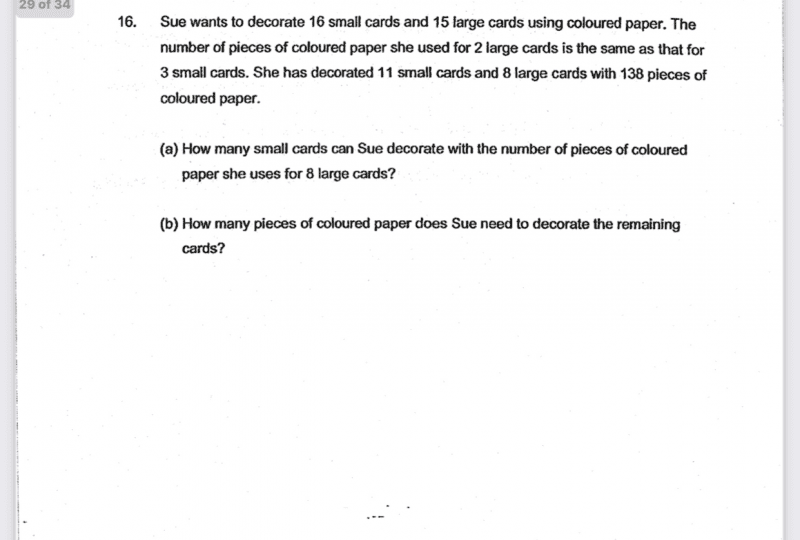QuestionI can only solve this with algebra.  Can someone do this with models, please?

Let S be the number of coloured paper needed for 1 small card

Let L be the number of coloured paper needed for 1 large card

2L = 3S

=> 3S – 2L = 0

(1) 12S – 8L = 0

(2) 11S + 8L = 138

(1)+(2): 23S = 138

So S = 6

Therefore L = 3S/2 = 9

(a) 8 large cards require 8L pieces of paper or 8*9 = 72

With the same amount of paper,

Number of small cards she can decorate is 72/6 = 12 #

(b) Paper for remaining cards are 5S + 7L = 5(6) + 7(9) = 30 + 63 = 93 #

0 Replies 1 Like